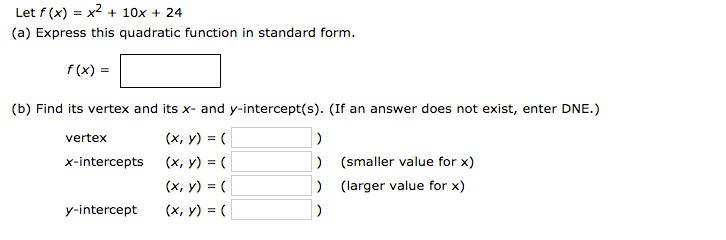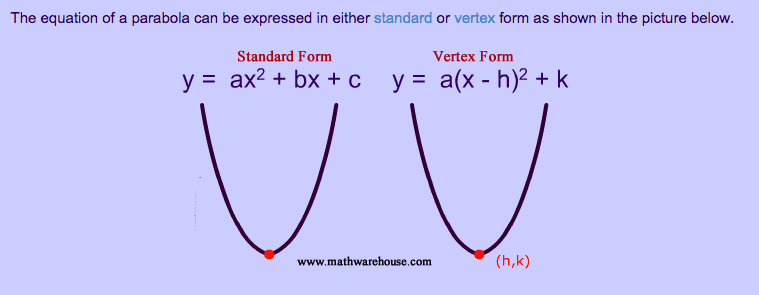# Standard form, vertex form. Something isn't right here

I mainly just need some clarification here. I was doing my homework and then browsing the web to find an answer to my problem and came across mathewarehouse' definition of Standard form and then I looked at my homework and went...."huh?" I don't understand if my homework is listening this wrong or mathwarehouse or if I am misunderstanding something.

Here are the two images my hw first:here it is telling me f(x)=x^2+10x+24 is a quadratic function which must be expressed in standard form. But mathewarehouse would leads me to think that it's already in standard form:What's the deal?

Mark44
Mentor
Looks like f is already in standard form.

The standard form is expressed by completing the square.

The vertex is simply the point on the parabola where the graph changes direction, as shown on the graph you posted above, the (h,k) coordinate which is determined from the vertex form equation (complete the square equation of the function).

I still don't understand. These two things are giving me conflicting ideas. Which is which? Is f(x)=x^2+10x+24 a quadratic function or is standard form? So maybe it's just trying to confuse me? and I just need to put it in twice and then to find the vertex but completing the square aka putting it in vertex form? is that right?

An equation can be expressed in standard or vertex form. A quadratic function in standard form is f(x) = a(x - h)2 + k.

It's just semantics really, albeit confusingly laid out above.

The standard and vertex equations ( y =) are referred to as standard and vertex respectively and in that sense are not incorrect in what is being shown, however, a quadratic function in standard form is expressed as f(x) = a(x - h)2 + k.

So in the question you are being asked to express a function in standard form, you complete the square. As with most mathematical questions a follow on effect is used, your answer for (a) is guiding you towards the answer for (b).

@Jimmy Johnson THANKS A TON! that clears up a lot. This math program I am using likes to be purposely cryptic I think.

Mark44
Mentor
You need to use whatever definition your program is using for the term "standard form." I don't think many textbooks would call y = a(x - h)2 + k "standard form" but your program seems to be doing this.

Homework Helper
No - standard form is the $ax^2 + bx + c$ version, and $a\left(x-h\right)^2 + k$ is the vertex form. Your text is using the names in a non-standard way - as is #5.

Mark44
Mentor
No - standard form is the $ax^2 + bx + c$ version, and $a\left(x-h\right)^2 + k$ is the vertex form. Your text is using the names in a non-standard way - as is #5.
I agree.

In most cases of this topic I've seen in textbooks or online the standard form of a quadratic function is expressed in vertex form. However I agree that it is inconsistent throughout different teachings etc.

"Different textbooks have different interpretations of the reference "standard form" of a quadratic function. Some say f (x) = ax2 + bx + c is "standard form", while others say that f (x) = a(x - h)2 + k is "standard form". To avoid confusion, this site will not refer to either as "standard form", but will reference f (x) = a(x - h)2 + k as "vertex form" and will reference f(x) = ax2 + bx + c by its full statement."

Nevertheless I think we can all agree that the standard form of this question is looking for the vertex form.

Ray Vickson
Homework Helper
Dearly Missed
In most cases of this topic I've seen in textbooks or online the standard form of a quadratic function is expressed in vertex form. However I agree that it is inconsistent throughout different teachings etc.

"Different textbooks have different interpretations of the reference "standard form" of a quadratic function. Some say f (x) = ax2 + bx + c is "standard form", while others say that f (x) = a(x - h)2 + k is "standard form". To avoid confusion, this site will not refer to either as "standard form", but will reference f (x) = a(x - h)2 + k as "vertex form" and will reference f(x) = ax2 + bx + c by its full statement."

Nevertheless I think we can all agree that the standard form of this question is looking for the vertex form.

No, I don't think we can agree on that. Perhaps the book (or on-line course, or whatever) really does mean that a*x^2 + b*x+c is the standard form, and maybe the question is merely asking the student to recognize that the given function is already in standard form. Sometimes simple exercises like that are given to help firm up a concept in a student's mind, the theory being that repetition helps. However, I would hope that somewhere in the course notes or lectures (or whatever) the concepts of "standard form" and "vertex form" are actually defined.

Mark44
Mentor
No, I don't think we can agree on that. Perhaps the book (or on-line course, or whatever) really does mean that a*x^2 + b*x+c is the standard form, and maybe the question is merely asking the student to recognize that the given function is already in standard form.
That was my thought, as well.
Ray Vickson said:
Sometimes simple exercises like that are given to help firm up a concept in a student's mind, the theory being that repetition helps. However, I would hope that somewhere in the course notes or lectures (or whatever) the concepts of "standard form" and "vertex form" are actually defined.

SammyS
Staff Emeritus
Homework Helper
Gold Member
In most cases of this topic I've seen in textbooks or online the standard form of a quadratic function is expressed in vertex form. However I agree that it is inconsistent throughout different teachings etc.

"Different textbooks have different interpretations of the reference "standard form" of a quadratic function. Some say f (x) = ax2 + bx + c is "standard form", while others say that f (x) = a(x - h)2 + k is "standard form". To avoid confusion, this site will not refer to either as "standard form", but will reference f (x) = a(x - h)2 + k as "vertex form" and will reference f(x) = ax2 + bx + c by its full statement."

Nevertheless I think we can all agree that the standard form of this question is looking for the vertex form.